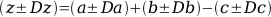# Science 2008

## Centrifuge RPM and RCF Calculator

This calculator allows you to inter-convert between RPM and RCF for any centrifuge with a known rotor size. There is a limited list of rotors below for manual rotor selection.

Variable to solve for Value Units
Rotational speed RPM
G-force (RCF) xg

You are currently solving for rotational speed (RPM)

## Standard Deviations

The correct manipulation of standard deviations is an often overlooked skill. When working with data sets it is cruicial that the correct manipluations are applied for the results to be valid.

This page demonstrates several methods for combining standard deviations correctly with some worked examples.

### Addition and subtraction of values with standard deviations

Consider this problem where each value (a, b or c) has an associated standard deviation or error (Da, Db or Dc respectively). We are trying to calculate the correct value for z ± Dz: